Share filterDeveloped by in Education category

4/5.0 Ratings

500,000 - 1,000,000 Installs

4,547 Reviews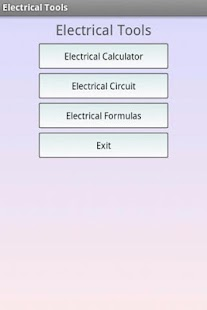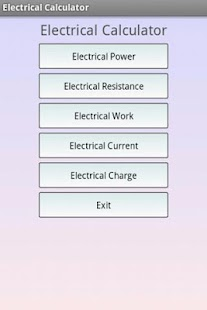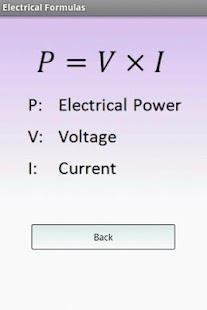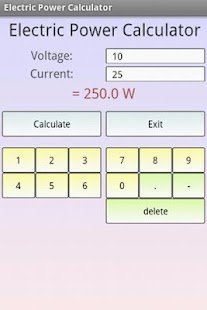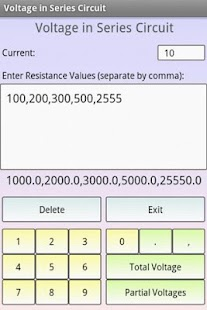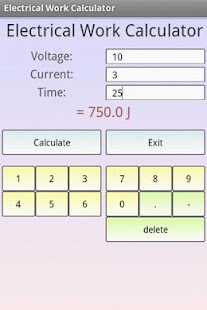### Description

This free app consists of 3 useful electricity tools, an electrical calculator, an electrical circuit calculator and electrical formulas.

Electrical calculator: You are able to calculate the most important electrical sizes. You can calculate the electrical power, electrical resistance, electrical charge, electrical work and electrical current.

Electrical circuit: You are able to calculate the current in parallel circuits (both total and partial), the voltage in series circuits (both total and partial) and the resistance in parallel and series circuits.

Electrical formulas: You can see the formulas for the electrical power, electrical resistance, electrical work, electrical current and electrical charge.

Electrical engineering Books: You can see a list of the best books and buy them.

Electrical engineering E-Books: You can see a list of the best e-books and buy them.

Other calculators: You can also calculate the battery lifetime, the stored energy in a capacitor, the inductance and the capacitance, the output voltage of a voltage divider.

The best app for school, college and work! If you are a student it will helps to learn.

Note: Electrical engineering covers a wide range of subfields including electronics, digital computers, power engineering, telecommunications, control systems, radio frequency engineering, and signal processing.

This content has been crawled through Play Store, we don't store any APK file.